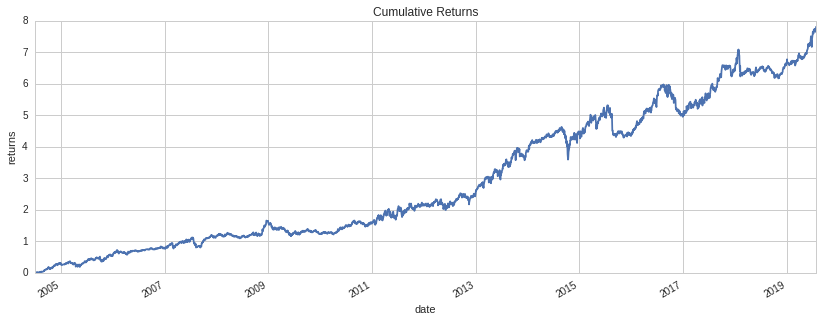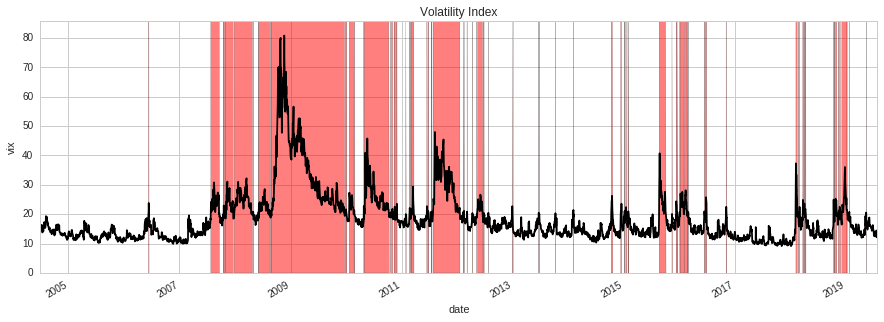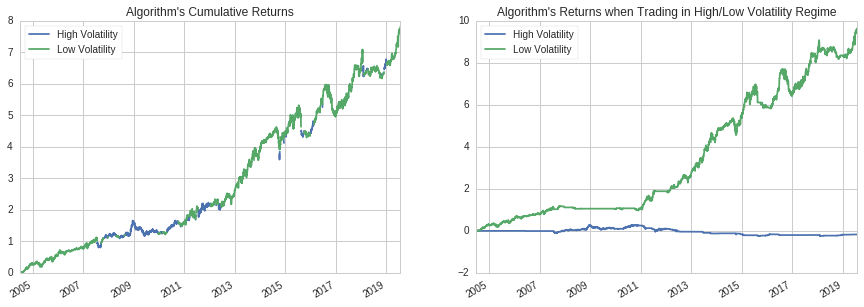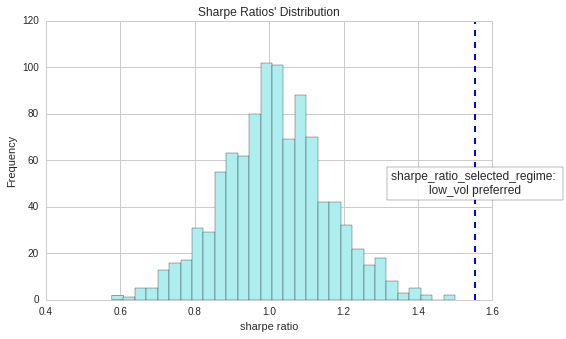Notebook

Measuring an algorithm’s sensitivity to volatility regimes

In :
# Load packages.
import pandas as pd
import numpy as np
import scipy.stats as st
import matplotlib.pyplot as plt
import empyrical as ep

In :
# Load backtest data.
bt = get_backtest('5d3f81bcd37324619924f177') # Replace the backtest_id with your own algo's backtest_id
returns = bt.daily_performance['returns']

# Get start date and end date for returns.
start_date = returns.index
end_date = returns.index[-1]

# print basic information about returns.
print "Earlest algo start date: {}.".format(start_date)
print "Latest algo end date: {}.".format(end_date)
print "Numbers of trading days: {}.".format(len(returns.index))

100% Time: 0:00:31|###########################################################|
Earlest algo start date: 2004-07-01 00:00:00+00:00.
Latest algo end date: 2019-07-26 00:00:00+00:00.


## Plot algo's cumulative returns¶

In :
cum_returns = ep.cum_returns(returns)
ax = cum_returns.plot(figsize=(14,5))
ax.set(title='Cumulative Returns', ylabel='returns', xlabel='date');In :
# Load VIX data (https://www.quantopian.com/data/quandl/cboe_vix)# Load
from quantopian.interactive.data.quandl import cboe_vix
from odo import odo

vix_data = (odo(cboe_vix[['vix_close', 'asof_date']], pd.DataFrame)
.set_index('asof_date')
.rename_axis('date')
.tz_localize('utc')
.sort_index()
.loc[start_date:end_date]
.vix_close)

# Show the first five data points.

Out:
date
2004-07-01 00:00:00+00:00    15.20
2004-07-02 00:00:00+00:00    15.08
2004-07-06 00:00:00+00:00    16.25
2004-07-07 00:00:00+00:00    15.81
2004-07-08 00:00:00+00:00    16.20
Name: vix_close, dtype: float64

## Plot VIX index and highlight high volatility regime¶

In :
# Plot VIX timeseries# Plot
ax = vix_data.plot(color='black', figsize=(15, 5))

# The threshold for dividing high/low vol regimes is the long-term mean of S&P 500 volatility.
threshold = 20 # 18, 20

# Highlight regions of high volatility.
x = vix_data.index
ymax = vix_data.max() + 5
ax.fill_between(x, 0, ymax, where=vix_data > threshold, facecolor='red', alpha=0.5, interpolate=True)

ax.set_xlim([x, x[-1]])
ax.set_ylim([0, ymax])
ax.set(title='Volatility Index', ylabel='vix');In :
# Boolean series containing True for high vol regimes, and False for low-vol regimes.
regimes = (vix_data >= threshold)

Out:
date
2004-07-01 00:00:00+00:00    False
2004-07-02 00:00:00+00:00    False
2004-07-06 00:00:00+00:00    False
2004-07-07 00:00:00+00:00    False
2004-07-08 00:00:00+00:00    False
Name: vix_close, dtype: bool

## Compute algo's sharpe ratios in high volatility regime and low volatility regime¶

In :
def regime_sharpe_ratio(returns, regimes):
'''
Compute Sharpe ratios for different regimes.

Parameters
----------
returns : pd.Series[float]
Series containing daily algorithm returns.
regimes: pd.Series[bool]
Series containing True/False values indicating whether a given day was
high or low volatility.
'''
regime_sharpe_ratio = {}
if regimes.all():
raise ValueError(
"The reference data does not involve any low volatility dates."
"Please try to run the analysis with longer backtest period."
)

if (~regimes).all():
raise ValueError(
"The reference data does not involve any high volatility' dates."
"Please try to run the analysis with longer backtest period."
)

regime_sharpe_ratio['high_vol'] = ep.sharpe_ratio(returns.loc[regimes])
regime_sharpe_ratio['low_vol'] = ep.sharpe_ratio(returns.loc[~regimes])
return pd.Series(regime_sharpe_ratio)

In :
sharpe_ratios = regime_sharpe_ratio(returns, regimes)

# Compute Sharpe ratio over entire data range.
overall_sharpe_ratio = ep.sharpe_ratio(returns)

In :
print "Sharpe ratio in entire period: {}.".format(overall_sharpe_ratio)
print "Sharpe ratio in selected vol periods: {}.".format(sharpe_ratios.max())
print "Sharpe ratio in high vol periods: {}.".format(sharpe_ratios['high_vol'])
print "Sharpe ratio in low vol periods: {}.".format(sharpe_ratios['low_vol'])
print "Possible preference: {}".format(sharpe_ratios.argmax())

Sharpe ratio in entire period: 1.03544611812.
Sharpe ratio in selected vol periods: 1.55312974925.
Sharpe ratio in high vol periods: -0.203975408055.
Sharpe ratio in low vol periods: 1.55312974925.
Possible preference: low_vol


## Plot algo's cumulative returns in different regimes¶

In :
def plot_cumulative_returns_by_regime(returns, regimes):
"""Plot cumulative returns generated within each regime.
Parameters
----------
returns : pd.Series[float]
Timeseries of algorithm returns.
regimes : pd.Series[bool]
Boolean series indicating whether each day was high or low volatility.
"""
fig, (original_ax, split_ax) = plt.subplots(ncols=2, nrows=1, figsize=(15, 5))
cum_rets = ep.cum_returns(pd.DataFrame({
'Returns': returns,
'High Volatility': returns.where(regimes, 0),
'Low Volatility': returns.where(~regimes, 0),
}))

# Plot algo's cumulative returns on the left axis.
pd.concat([cum_rets['Returns'].where(regimes, np.nan).rename('High Volatility'),
cum_rets['Returns'].where(~regimes, np.nan).rename('Low Volatility')],
axis=1).plot(ax=original_ax, title="Algorithm's Cumulative Returns")

# Plot cumulative returns within each regime on the right axis.
title = "Algorithm's Returns when Trading in High/Low Volatility Regime"
cum_rets[['High Volatility', 'Low Volatility']].plot(ax=split_ax, title=title)

plot_cumulative_returns_by_regime(returns, regimes)## Run Bootstrap Test¶

In :
# function used for bootstrap: get random test dates.
def random_date_ranges(date_index, segments_lengths):
"""
Generate bootstrap samples with same segment numbers
and lengths over the entire data range.

Parameters
----------
date_index : pd.Series
The data range used for bootstrap test.
segments_lengths : list
The numbers of consecutive days in a selected high vol/low vol regime.

Returns
-------
test_dates_index : pd.Series
Index series for bootstrap.
"""

# Generate bootstrap random samples from the entire data range.
total_segments_length = sum(segments_lengths)
total_gaps_length = len(date_index) - total_segments_length
gaps_lengths = random_partition(total_gaps_length, len(segments_lengths) + 1)
# shuffle the segments' lengths of the selected regime
np.random.shuffle(segments_lengths)

samples = []
cursor = 0
for gap, segment in zip(gaps_lengths, segments_lengths):
cursor += gap
samples.append(date_index[cursor:cursor + segment])
cursor += segment
return pd.Index(np.hstack(samples), tz='UTC')

# function used for getting random test dates.
def random_partition(N, k):
"""
Randomly generate a partition of the integer N into k pieces.
"""
samples = np.sort(np.random.choice(N + 1, replace=False, size=k - 1))
lengths = np.hstack([samples, np.diff(samples), N - samples[-1]])
assert lengths.sum() == N, lengths.sum()

return lengths.tolist()

# function used for bootstrap: count consecutive days in high/low vol range.
def reference_regime_segments(selected_regime_data):
'''
Count consecutive days in each high/low vol range and store the number.

Parameters
----------
selected_regime_data : pd.Series
Referece data from a selected regime.

Returns
-------
segments_lengths : pd.Series
A series of numbers of consecutive days in a selected regime.
'''
lengths = (selected_regime_data.groupby((~selected_regime_data).cumsum())
.sum()
.astype(int))
# Remove possible leading and trailing zeros.
segment_lengths = list(lengths.loc[lengths != 0].values)
return segment_lengths

def compute_percentile_score(returns, selection, output=True, seed=0):
'''
Compute percentile score for selected Sharpe ratio in random Sharpe ratio distribution.

Parameters
----------
returns : pd.Series[float]
Timeseries of algorithm returns.
selection : pd.Series[bool]
Boolean series indicating whether each day was in the selected regime or not.
output :  bool (optional)
Whether to output the variables of sharpe_ratio_selected_regime and random_sharpe_ratios.
seed : int, optional
Seed to use for random number generation.

Returns
-------
score : float
The percentile score for selected Sharpe ratio.
sharpe_ratio_selected_regime : float
The Sharpe ratio of returns in the selected regime
random_sharpe_ratios:  pd.Series
Samples' sharpe ratios
'''
rng = np.random.RandomState(seed)
random_sharpe_ratios = []
num_samples = 1000

# Sharpe ratio of algorithm returns on selected days.
sharpe_ratio_selected_regime = ep.sharpe_ratio(returns.loc[selection])

# Compute the segments' lengths in the selected regime
segments_lengths = reference_regime_segments(selection)

for j in range(num_samples):
test_dates = random_date_ranges(returns.index, segments_lengths)
random_sharpe_ratios.append(ep.sharpe_ratio(returns.loc[test_dates]))

# compute the percentile of sharpe_ratio_selected_vol
# in Sharpe ratio distribution generated by bootstrapping.
score = st.percentileofscore(random_sharpe_ratios,
sharpe_ratio_selected_regime,
kind='strict')
if output:
random_sharpe_ratios = pd.Series(random_sharpe_ratios)
return score, sharpe_ratio_selected_regime, random_sharpe_ratios
else:
return score
return score

In :
if sharpe_ratios['high_vol'] >= sharpe_ratios['low_vol']:
print("Computing percentile scores for high vol regime...")
selection = regimes
else:
print("Computing percentile scores for low vol regime...")
selection = ~regimes
(score,
sharpe_ratio_selected_regime,
random_sharpe_ratios) = compute_percentile_score(returns, selection, output=True)
print "Done!"

Computing percentile scores for low vol regime...
Done!


## Plot the distribution of Sharpe ratios generated by bootstrap¶

In :
# Plot Sharpe ratio distribution and mark the sharpe_ratio_selected_regime position in the distribution.
ax = pd.Series(random_sharpe_ratios).plot.hist(bins=30, color='paleturquoise', figsize=(8,5))
ax.axvline(sharpe_ratio_selected_regime, color='b', linestyle='dashed', linewidth=2)

ax.text(sharpe_ratio_selected_regime,
ax.get_ylim()+50,
"sharpe_ratio_selected_regime: \n{}".format(sharpe_ratios.argmax()+' preferred'),
horizontalalignment='center',
verticalalignment='center',
bbox=dict(facecolor='white', alpha=0.9))
ax.set(title="Sharpe Ratios' Distribution", xlabel='sharpe ratio');## Summarize the algo's performance and its volatility preference.¶

In :
pd.Series({'algo_pattern' : sharpe_ratios.argmax()+' preferred',
'overall_sharpe_ratio' : overall_sharpe_ratio,
'sharpe_ratio_high_vol' : sharpe_ratios['high_vol'],
'sharpe_ratio_low_vol' : sharpe_ratios['low_vol'],
'sharpe_ratio_preferred_vol' : sharpe_ratios.max(),
'percentile_score': score}).to_frame('vol_regime_analysis')

Out:
vol_regime_analysis
algo_pattern low_vol preferred
overall_sharpe_ratio 1.03545
percentile_score 100
sharpe_ratio_high_vol -0.203975
sharpe_ratio_low_vol 1.55313
sharpe_ratio_preferred_vol 1.55313